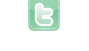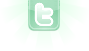# Confused

i have two actor 1.Tree and 2.squirrel .. i am creating two object of Tree and one object of squirrel. now squirrel is on one tree and i want to move it on another tree .(* both tree are linear )(squirrel move -5 and stop when tree found) if ( !isTouching(Tree.class)) { move(-5); }
Is the moving from one tree to the other a one-time thing and only from the tree on the right to the tree on the left?
my ultimate goal is to move squirrel from one tree to another no matter left or right. ( before develop random moving it should work for one to next tree that's why i want to move squirrel from right tree to left tree (* tree are of one class)).
Maybe if the squirrel knew where the midpoint was between the trees, it can be made to easily move from one tree to the other:
```private int midTrees = 0;
private int direction = 1;

public void act()
{
if (midTrees == 0)
{
for (Object obj : getWorld().getObjects(Tree.class)) midTrees += ((Actor)obj).getX();
midTrees = midTrees/2;
}
if (!isTouchhing(Tree.class) || (isTouching(Tree.class) && direction*(getX()-midTrees) < 0) move(direction*5);
else if (Greenfoot.getRandomNumber(600) == 0) direction = -direction;
}```
Thanks a lot , its working for now..